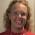Percentages with mental math

(This is an older post that I have revised plus added a video to it.)
In this article I want to explore some ideas for using MENTAL math in calculating percents or percentages.

And here are the ideas:
1. Find 10% of some example numbers (by dividing by 10).
2. Find 1% of some example numbers (by dividing by 100).
3. Find 20%, 30%, 40% etc. of these numbers.
FIRST find 10% of the number, then multiply by 2, 3, 4, etc.
For example, find 20% of 18. Find 40% of \$44. Find 80% of 120.

I know you can teach the student to go 0.2 × 18, 0.4 × 0.44, and 0.8 × 120 - however when using mental math, the above method seems to me to be more natural.
4. Find 3%, 4%, 6% etc. of these numbers.
FIRST find 1% of the number, then multiply.
5. Find 15% of some numbers.
First find 10%, halve that to find 5%, and add the two results.
6. Find 25% and then 75% of some numbers. 25% of a number is 1/4 of it, so you find it by dividing by 4. For example, 25% of 16 is 4. To find 75%, first find 25% and multiply that by 3.
7. Calculate some simple discounts. If an item is discounted 20%, 15%, 25%, 75% etc., then find the new price.

For example, a book costs \$40 and is discounted by 15%. What is the new price?
First find 15% of \$40 (10% of \$40 is \$4 and 5% of \$40 is \$2... so 15% of it is \$6). Then subtract \$40 - \$6. So the new price is \$34.
8. "40% of a number is 56. What is the number?" - types of problems.

You CAN do this mentally: First FIND 10% of the number, and then multiply that result by 10, and you'll get 100% of the number - which is the number itself.

So if 40% is 56, then 10% is 14 (divide by 4). Then, 100% of the number is 140. This result is reasonable, because 40% of this number was 56, so the actual number (140) needs to be more than double that.
9. "34% of a number is 129. What is the number?" (A calculator will help here.)

You don't need to write an equation. You can just first find 1% of this number, and then find 100% of the number.

If 34% of a number is 129, then 1% of that number is 129/34. Find that, and multiply the result by 100.

Hope you enjoyed these little mental math ideas! They also help students understand the concept of percent where they don't end up relying too much on mechanical calculations or equations.Beckie Russell said…
Finding 75%: I like to find 25% by dividing by 4 and then subtracting from the original amount. It's a nice use of the complement and is easier, I think, than multiplying by 3. I do it with large percentages that are multiples of 10, too. E.G. 80% = original amount - 20%.Anonymous said…
great video, very good explanationsdebra said…
do you have any more video's of percents?Maria Miller said…
At the moment I don't have other videos about percent, but I'm sure I will later. However, I have written articles about percent that might be of help.Vic Piercy said…
Great video, easy to understand explanations. Thank you!AprilS said…
We just posted a blog post on our site about Percents. It includes free video from our 6th grade math curriculum:

http://blog.thinkwell.com/2010/06/percents.html